This is “Solving Linear Inequalities with One Variable”, section 1.8 from the book Advanced Algebra (v. 1.0). For details on it (including licensing), click here.

Has this book helped you? Consider passing it on:
Creative Commons supports free culture from music to education. Their licenses helped make this book available to you.
DonorsChoose.org helps people like you help teachers fund their classroom projects, from art supplies to books to calculators.

## 1.8 Solving Linear Inequalities with One Variable

### Learning Objectives

1. Identify linear inequalities and check solutions.
2. Solve linear inequalities and express the solutions graphically on a number line and in interval notation.
3. Solve compound linear inequalities and express the solutions graphically on a number line and in interval notation.
4. Solve applications involving linear inequalities and interpret the results.

## Linear Inequalities

A linear inequalityLinear expressions related with the symbols $≤$, <, $≥$, and >. is a mathematical statement that relates a linear expression as either less than or greater than another. The following are some examples of linear inequalities, all of which are solved in this section:

 $5x+7<22$ $−2(x+8)+6≥20$ $−2(4x−5)<9−2(x−2)$

A solution to a linear inequalityA real number that produces a true statement when its value is substituted for the variable. is a real number that will produce a true statement when substituted for the variable. Linear inequalities have either infinitely many solutions or no solution. If there are infinitely many solutions, graph the solution set on a number line and/or express the solution using interval notation.

### Example 1

Are $x=−4$ and $x=6$ solutions to $5x+7<22$?

Solution:

Substitute the values in for x, simplify, and check to see if we obtain a true statement.

Check $x=−4$

Check $x=6$

$5(−4)+7<22−20+7<22−13<22 ✓$

$5(6)+7<2230+7<2237<22 ✗$

Answer: $x=−4$ is a solution and $x=6$ is not.

All but one of the techniques learned for solving linear equations apply to solving linear inequalities. You may add or subtract any real number to both sides of an inequality, and you may multiply or divide both sides by any positive real number to create equivalent inequalities. For example:

$10>−510−7>−5−7 Subtract 7 on both sides.3>−12 ✓ True$

$10>−5105>−55 Divide both sides by 5.2>−1 ✓True$

Subtracting 7 from each side and dividing each side by positive 5 results in an inequality that is true.

### Example 2

Solve and graph the solution set: $5x+7<22.$

Solution:

$5x+7<225x+7−7<22−75x<155x5<155x<3$It is helpful to take a minute and choose a few values in and out of the solution set, substitute them into the original inequality, and then verify the results. As indicated, you should expect $x=0$ to solve the original inequality and that $x=5$ should not.

Check $x=0$

Check $x=5$

$5(0)+7<227<22 ✓$

$5(5)+7<2225+7<2232<22 ✗$

Checking in this manner gives us a good indication that we have solved the inequality correctly.

We can express this solution in two ways: using set notation and interval notation.

${x|x<3} Set notation (−∞,3) Interval notation$

In this text we will choose to present answers using interval notation.

Answer: $(−∞, 3)$

When working with linear inequalities, a different rule applies when multiplying or dividing by a negative number. To illustrate the problem, consider the true statement $10>−5$ and divide both sides by −5.

$10>−510−5>−5−5 Divide both sides by −5.−2>1 ✗ False$

Dividing by −5 results in a false statement. To retain a true statement, the inequality must be reversed.

$10>−510−5<−5−5 Reverse the inequality.−2<1 ✓True$

The same problem occurs when multiplying by a negative number. This leads to the following new rule: when multiplying or dividing by a negative number, reverse the inequality. It is easy to forget to do this so take special care to watch for negative coefficients. In general, given algebraic expressions A and B, where c is a positive nonzero real number, we have the following properties of inequalitiesProperties used to obtain equivalent inequalities and used as a means to solve them.:

 Addition property of inequalities: $If A Subtraction property of inequalities: $If A Multiplication property of inequalities: $If A $If A−cB$ Division property of inequalities: $If A $If AB−c$

We use these properties to obtain an equivalent inequalityInequalities that share the same solution set., one with the same solution set, where the variable is isolated. The process is similar to solving linear equations.

### Example 3

Solve and graph the solution set: $−2(x+8)+6≥20.$

Solution:

$−2(x+8)+6≥20 Distribute.−2x−16+6≥20 Combine like terms.−2x−10≥20 Solve for x.−2x≥30 Divide both sides by −2.−2x−2≤30−2 Reverse the inequality.x≤−15$Answer: Interval notation $(−∞, −15]$

### Example 4

Solve and graph the solution set: $−2(4x−5)<9−2(x−2).$

Solution:

$−2(4x−5)<9−2(x−2)−8x+10<9−2x+4−8x+10<13−2x−6x<3−6x−6>3−6 Reverse the inequality.x>−12$Answer: Interval notation $(−12, ∞)$

### Example 5

Solve and graph the solution set: $12x−2≥12(74x−9)+1.$

Solution:

$12x−2≥12(74x−9)+1 12x−2≥78x−92+1 12x−78x≥−72+2−38x≥−32 (−83)(−38x)≤(−83)(−32) Reverse the inequality. x≤4$Answer: Interval notation: $(−∞, 4]$

Try this! Solve and graph the solution set: $10−5(2x+3)≤25.$

Answer: $[−3, ∞)$;## Compound Inequalities

Following are some examples of compound linear inequalities:

 $−13<3x−7<17$ $4x+5≤−15 or 6x−11>7$

These compound inequalitiesTwo or more inequalities in one statement joined by the word “and” or by the word “or.” are actually two inequalities in one statement joined by the word and or by the word or. For example, $−13<3x−7<17$ is a compound inequality because it can be decomposed as follows: $−13<3x−7 and 3x−7<17$

We can solve each inequality individually; the intersection of the two solution sets solves the original compound inequality. While this method works, there is another method that usually requires fewer steps. Apply the properties of this section to all three parts of the compound inequality with the goal of isolating the variable in the middle of the statement to determine the bounds of the solution set.

### Example 6

Solve and graph the solution set: $−13<3x−7<17.$

Solution:

$−13<3x−7<17−13 +7<3x−7 +7<17 +7−6<3x<24−63<3x3<243−2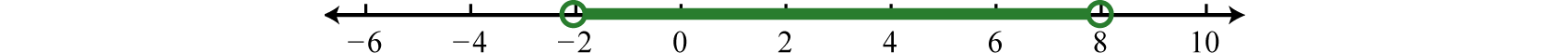Answer: Interval notation: $(−2,8)$

### Example 7

Solve and graph the solution set: $56≤13(12x+4)<2.$

Solution:

$56≤13(12x+4)<256≤16x+43<26⋅(56)≤6⋅(16x+43)<6⋅(2)5≤x+8<125−8≤x+8−8<12−8−3≤x<4$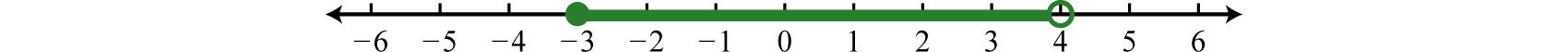Answer: Interval notation $[−3,4)$

It is important to note that when multiplying or dividing all three parts of a compound inequality by a negative number, you must reverse all of the inequalities in the statement. For example: $−10<−2x<20−10−2>−2x−2>20−25> x >−10$ The answer above can be written in an equivalent form, where smaller numbers lie to the left and the larger numbers lie to the right, as they appear on a number line. $−10 Using interval notation, write: $(−10, 5).$

Try this! Solve and graph the solution set: $−3≤−3(2x−3)<15.$

Answer: $(−1,2]$;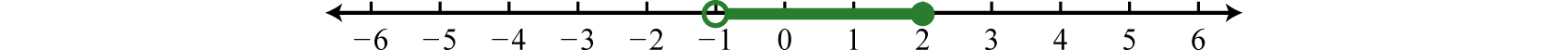For compound inequalities with the word “or” you work both inequalities separately and then consider the union of the solution sets. Values in this union solve either inequality.

### Example 8

Solve and graph the solution set: $4x+5≤−15 or 6x−11>7.$

Solution:

Solve each inequality and form the union by combining the solution sets.

 $4x+5≤−154x≤−20x≤−5$ or $6x−11>76x>18x>3$Answer: Interval notation $(−∞,−5]∪(3,∞)$

Try this! Solve and graph the solution set: $5(x−3)<−20 or 2(5−3x)<1.$

Answer: $(−∞,−1)∪(32, ∞)$;## Applications of Linear Inequalities

Some of the key words and phrases that indicate inequalities are summarized below:

Key Phrases

Translation

A number is at least 5.

$x≥5$

A number is 5 or more inclusive.

A number is at most 3.

$x≤3$

A number is 3 or less inclusive.

A number is strictly less than 4.

$x<4$

A number is less than 4, noninclusive.

A number is greater than 7.

$x>7$

A number is more than 7, noninclusive.

A number is in between 2 and 10.

$2

A number is at least 5 and at most 15.

$5≤x≤15$

A number may range from 5 to 15.

As with all applications, carefully read the problem several times and look for key words and phrases. Identify the unknowns and assign variables. Next, translate the wording into a mathematical inequality. Finally, use the properties you have learned to solve the inequality and express the solution graphically or in interval notation.

### Example 9

Seven less than 3 times the sum of a number and 5 is at most 11. Find all numbers that satisfy this condition.

Solution:

First, choose a variable for the unknown number and identify the key words and phrases.

Let n represent the unknown indicated by “a number.”Solve for n.

$3(n+5)−7≤113n+15−7≤113n+8≤113n≤3n≤1$

Answer: Any number less than or equal to 1 will satisfy the statement.

### Example 10

To earn a B in a mathematics course the test average must be at least 80% and less than 90%. If a student earned 92%, 96%, 79%, and 83% on the first four tests, what must she score on the fifth test to earn a B?

Solution:

Set up a compound inequality where the test average is between 80% and 90%. In this case, include the lower bound, 80.

Let x represent the score on the fifth test.

$80≤test average<9080≤92+96+79+83+x5<905⋅80≤5⋅350+x5<5⋅90400≤350+x<45050≤x<100$

Answer: She must earn a score of at least 50% and less than 100%.

In the previous example, the upper bound 100% was not part of the solution set. What would happen if she did earn a 100% on the fifth test?

$average=92+96+79+83+1005=4505=90$

As we can see, her average would be 90%, which would earn her an A.

### Key Takeaways

• Inequalities typically have infinitely many solutions. The solutions are presented graphically on a number line or using interval notation or both.
• All but one of the rules for solving linear inequalities are the same as solving linear equations. If you divide or multiply an inequality by a negative number, reverse the inequality to obtain an equivalent inequality.
• Compound inequalities involving the word “or” require us to solve each inequality and form the union of each solution set. These are the values that solve at least one of the given inequalities.
• Compound inequalities involving the word “and” require the intersection of the solution sets for each inequality. These are the values that solve both or all of the given inequalities.
• The general guidelines for solving word problems apply to applications involving inequalities. Be aware of a new list of key words and phrases that indicate a mathematical setup involving inequalities.

### Part A: Linear Inequalities

Determine whether or not the given value is a solution.

1. $5x−1<−2; x=−1$

2. $−3x+1>−10; x=1$

3. $2x−3<−5; x=1$

4. $5x−7<0; x=2$

5. $9y−4≥5$;   $y=1$

6. $−6y+1≤3$;   $y=−1$

7. $12a+3≤−2$;   $a=−13$

8. $25a−2≤−22$;   $a=−45$

9. $−10<2x−5<−5$;   $x=−12$

10. $3x+8<−2 or 4x−2>5$;   $x=2$

Graph all solutions on a number line and provide the corresponding interval notation.

1. $3x+5>−4$

2. $2x+1>−1$

3. $5−6y<−1$

4. $7−9y>43$

5. $6−a≤6$

6. $−2a+5>5$

7. $5x+63≤7$

8. $4x+116≤12$

9. $12y+54≥14$

10. $112y+23≤56$

11. $2(3x+14)<−2$

12. $5(2y+9)>−15$

13. $5−2(4+3y)≤45$

14. $−12+5(5−2x)<83$

15. $6(7−2a)+6a≤12$

16. $2a+10(4−a)≥8$

17. $9(2t−3)−3(3t+2)<30$

18. $−3(t−3)−(4−t)>1$

19. $12(5x+4)+56x>−43$

20. $25+16(2x−3)≥115$
21. $5x−2(x−3)<3(2x−1)$

22. $3(2x−1)−10>4(3x−2)−5x$

23. $−3y≥3(y+8)+6(y−1)$

24. $12≤4(y−1)+2(2y+1)$

25. $−2(5t−3)−4>5(−2t+3)$

26. $−7(3t−4)>2(3−10t)−t$

27. $12(x+5)−13(2x+3)>76x+32$
28. $−13(2x−3)+14(x−6)≥112x−34$
29. $4(3x+4)≥3(6x+5)−6x$

30. $1−4(3x+7)<−3(x+9)−9x$

31. $6−3(2a−1)≤4(3−a)+1$

32. $12−5(2a+6)≥2(5−4a)−a$

### Part B: Compound Inequalities

Graph all solutions on a number line and provide the corresponding interval notation.

1. $−1<2x+1<9$

2. $−4<5x+11<16$

3. $−7≤6y−7≤17$

4. $−7≤3y+5≤2$

5. $−7<3x+12≤8$

6. $−1≤2x+73<1$

7. $−4≤11−5t<31$

8. $15<12−t≤16$

9. $−13≤16a+13≤12$
10. $−16<13a+56<32$
11. $5x+2<−3​ or 7x−6>15$

12. $4x+15≤−1​ or 3x−8≥−11$

13. $8x−3≤1​ or 6x−7≥8$

14. $6x+1<−3​ or 9x−20>−5$

15. $8x−7<1​ or 4x+11>3$

16. $10x−21<9​ or 7x+9≥30$

17. $7+2y<5​ or 20−3y>5$

18. $5−y<5 or 7−8y≤23$

19. $15+2x<−15 or 10−3x>40$

20. $10−13x≤5 or 5−12x≤15$

21. $9−2x≤15​ and 5x−3≤7$

22. $5−4x>1 and 15+2x≥5$

23. $7y−18<17 and 2y−15<25$

24. $13y+20≥7 and 8+15y>8$

25. $5−4x≤9 and 3x+13≤1$

26. $17−5x≥7 and 4x−7>1$

27. $9y+20≤2 and 7y+15≥1$

28. $21−6y≤3 and −7+2y≤−1$

29. $−21<6(x−3)<−9$

30. $0≤2(2x+5)<8$

31. $−15≤5+4(2y−3)<17$

32. $5<8−3(3−2y)≤29$

33. $5<5−3(4+t)<17$

34. $−3≤3−2(5+2t)≤21$

35. $−40<2(x+5)−(5−x)≤−10$

36. $−60≤5(x−4)−2(x+5)≤15$

37. $−12<130(x−10)<13$
38. $−15≤115(x−7)≤13$
39. $−1≤a+2(a−2)5≤0$
40. $0<5+2(a−1)6<2$

### Part C: Applications

Find all numbers that satisfy the given condition.

1. Three less than twice the sum of a number and 6 is at most 13.

2. Five less than 3 times the sum of a number and 4 is at most 10.

3. Five times the sum of a number and 3 is at least 5.

4. Three times the difference between a number and 2 is at least 12.

5. The sum of 3 times a number and 8 is between 2 and 20.

6. Eight less than twice a number is between −20 and −8.

7. Four subtracted from three times some number is between −4 and 14.

8. Nine subtracted from 5 times some number is between 1 and 11.

Set up an algebraic inequality and then solve.

1. With a golf club membership, costing $120 per month, each round of golf costs only$35.00. How many rounds of golf can a member play if he wishes to keep his costs $270 per month at most? 2. A rental truck costs$95 per day plus $0.65 per mile driven. How many miles can be driven on a one-day rental to keep the cost at most$120?

3. Mark earned 6, 7, and 10 points out of 10 on the first three quizzes. What must he score on the fourth quiz to average at least 8?

4. Joe earned scores of 78, 82, 88 and 70 on his first four algebra exams. What must he score on the fifth exam to average at least 80?

5. A gymnast scored 13.2, 13.0, 14.3, 13.8, and 14.6 on the first five events. What must he score on the sixth event to average at least 14.0?

6. A dancer scored 7.5 and 8.2 from the first two judges. What must her score from the third judge come in as if she is to average 8.4 or higher?

7. If two times an angle is between 180 degrees and 270 degrees, then what are the bounds of the original angle?

8. The perimeter of a square must be between 120 inches and 460 inches. Find the length of all possible sides that satisfy this condition.

9. A computer is set to shut down if the temperature exceeds 45°C. Give an equivalent statement using degrees Fahrenheit. Hint: $C=59(F−32).$

10. A certain antifreeze is effective for a temperature range of −35°C to 120°C. Find the equivalent range in degrees Fahrenheit.

### Part D: Discussion Board

1. Often students reverse the inequality when solving $5x+2<−18$? Why do you think this is a common error? Explain to a beginning algebra student why we do not.

2. Conduct a web search for “solving linear inequalities.” Share a link to website or video tutorial that you think is helpful.

3. Write your own 5 key takeaways for this entire chapter. What did you find to be review and what did you find to be new? Share your thoughts on the discussion board.

1. Yes

2. No

3. Yes

4. No

5. Yes

6. $(−3,∞)$;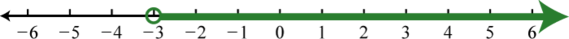7. $(1,∞)$;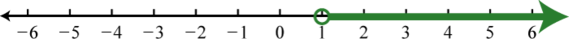8. $[0,∞)$;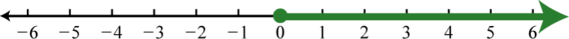9. $(−∞,3]$;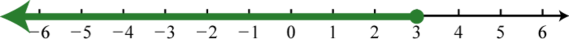10. $[−2,∞)$;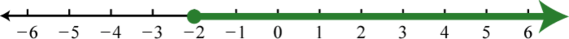11. $(−∞,−5)$;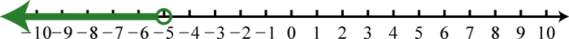12. $[−8,∞)$;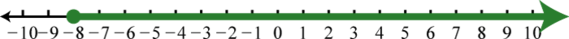13. $[5,∞)$;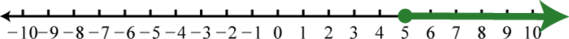14. $(−∞,7)$;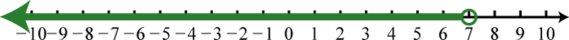15. $(−1,∞)$;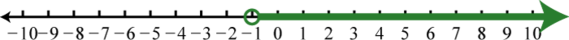16. $(3,∞)$;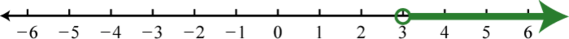17. $(−∞,−32]$;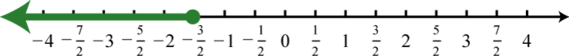18. Ø;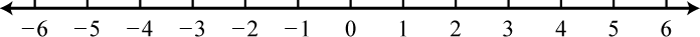19. $(−∞,0)$;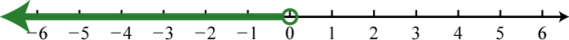20. $ℝ$;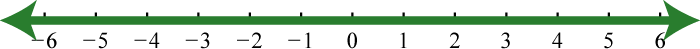21. $[−2,∞)$;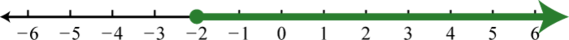1. $(−1,4)$;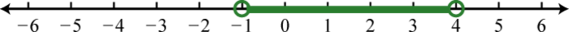2. $[0,4]$;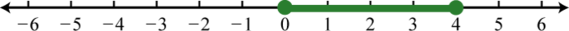3. $(−5,5]$;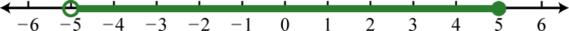4. $(−4,3]$;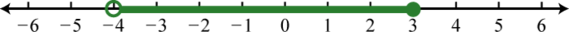5. $[−4,1]$;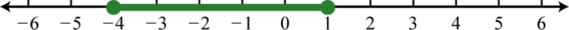6. $(−∞,−1)∪(3,∞)$;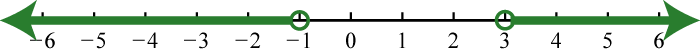7. $(−∞,12]∪[52,∞)$;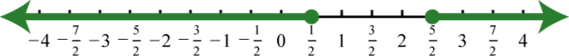8. $ℝ$;9. $(−∞,5)$;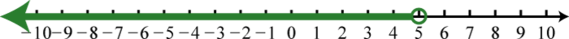10. $(−∞,−10)$;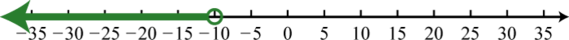11. $[−3,2]$;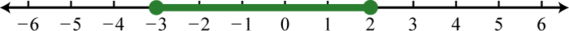12. $(−∞,5)$;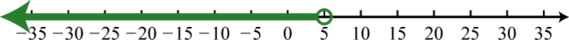13. Ø;14. −2;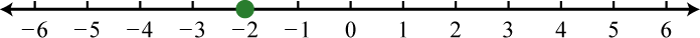15. $(−12,32)$;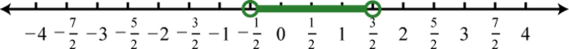16. $[−1,3)$;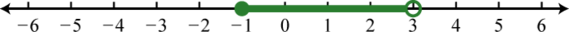17. $(−8,−4)$;18. $(−15,−5]$;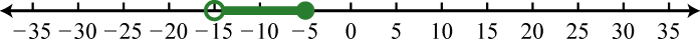19. $(−5,20)$;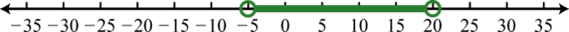20. $[−13, 43]$;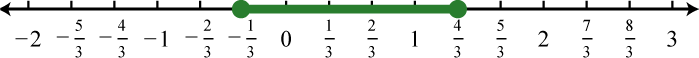1. $(−∞,2]$

2. $[−2,∞)$

3. $(−2,4)$

4. $(0,6)$

5. Members may play 4 rounds or fewer.

6. Mark must earn at least 9 points on the fourth quiz.

7. He must score a 15.1 on the sixth event.

8. The angle is between 90 degrees and 135 degrees.

9. The computer will shut down when the temperature exceeds 113°F.